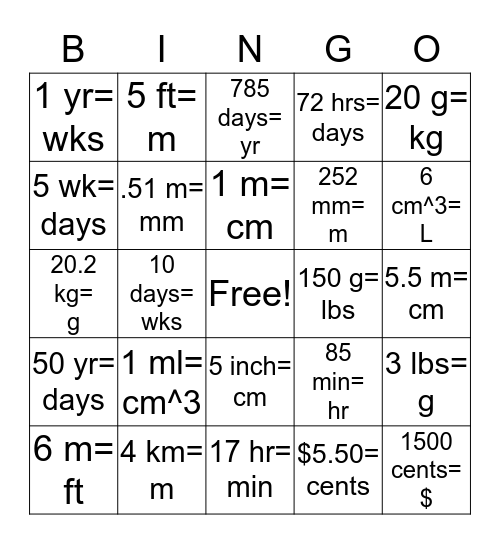Unit Conversion BingoThis bingo card has a free space and 24 words: 1 ml= cm^3, 4 km= m, 1 m= cm, 6 m= ft, 20 g= kg, 1 yr= wks, 5 wk= days, 5 ft= m, 150 g= lbs, 5 inch= cm, 252 mm= m, 20.2 kg= g, 85 min= hr, 3 lbs= g, .51 m= mm, \$5.50= cents, 5.5 m= cm, 785 days= yr, 6 cm^3= L, 10 days= wks, 1500 cents= \$, 50 yr= days, 72 hrs= days and 17 hr= min.

Play Online